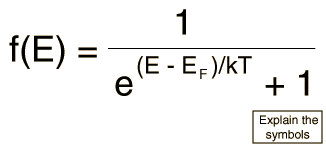# Fermi-dirac distribution

• magnifik

#### magnifik

An electron state has energy 0.14 eV above the Fermi energy. What is the probability that the electron state will be occupied at T = 200K?

do i just use the following formula?the part that throws me off is the "above the Fermi energy" bit. would i just plug that number in for E - Ef??

Yep, it means that E = EF + 0.14 eV, so E - EF = 0.14 eV.